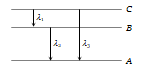# NEET Physics Atoms Questions Solved

Energy levels A, B, C of a certain atom corresponding to increasing values of energy i.e. ${\mathrm{E}}_{\mathrm{A}}<{\mathrm{E}}_{\mathrm{B}}<{\mathrm{E}}_{\mathrm{C}}$. If  are the wavelengths of radiations corresponding to the transitions C to B, B to A and C to A respectively, which of the following statements is correct(a) ${\mathrm{\lambda }}_{3}={\mathrm{\lambda }}_{1}+{\mathrm{\lambda }}_{2}$                      (b) ${\mathrm{\lambda }}_{3}=\frac{{\mathrm{\lambda }}_{1}{\mathrm{\lambda }}_{2}}{{\mathrm{\lambda }}_{1}+{\mathrm{\lambda }}_{2}}$
(c) ${\mathrm{\lambda }}_{1}+{\mathrm{\lambda }}_{2}+{\mathrm{\lambda }}_{3}=0$                 (d) ${\mathrm{\lambda }}_{3}^{2}={\mathrm{\lambda }}_{1}^{2}+{\mathrm{\lambda }}_{2}^{2}$